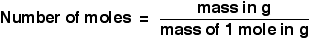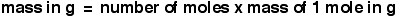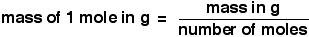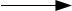Chemguide: Support for CIE A level Chemistry ``` ``` Learning outcome 2.2 The mole and the Avogadro constant ``` ``` Learning outcome 2.2.1 This statement deals with the mole concept and the Avogadro Constant. Before you go on, you should find and read the statement in your copy of the syllabus. For the examples which follow on this page, you will also need to refer to the Periodic Table towards the end of the syllabus to find the relative atomic masses of the elements. It would probably pay you to print that page. ``` ``` Working with moles I am going to start by explaining how you use moles rather than the reasoning behind them. Using them is easy, and you will have to be really comfortable about doing it, otherwise you won't be able to do most chemistry sums. Once you are confident about this, we can worry about the logic behind them. ``` ``` Working out the mass of a mole of a substance Easy! Work out the relative formula mass, and then add the unit "grams". Example 1: The mass of 1 mole of water, H2O The relative formula mass of water, H2O = (2 x 1) + 16 =18 1 mole of water, H2O, weighs 18 g. ``` ``` Example 2: The mass of 1 mole of sodium carbonate crystals, Na2CO3.10H2O The relative formula mass of sodium carbonate crystals, Na2CO3.10H2O = (2 x 23) + 12 + (3 x 16) + 10 x [(2 x 1) + 16] = 286 1 mole of sodium carbonate crystals, Na2CO3.10H2O, weighs 286 g ``` ``` The importance of quoting the formula Whenever you talk about a mole of something you must quote the formula. For example, if you were talking about a mole of sodium carbonate, you could mean a mole of anhydrous sodium carbonate, Na2CO3, or a mole of the crystals, Na2CO3.10H2O. The mass of 1 mole of the anhydrous compound is 106 g; the mass of a mole of the crystals is 286 g. Or if you were talking about the mass of a mole of oxygen, you might be talking about a mole of oxygen atoms, O, or a mole of oxygen molecules, O2. Always write down the formula you are referring to. ``` ``` Converting between the mass of a substance and the number of moles You need to practise this until you can do it without really thinking about it. The formula is this:So, if 1 mole of a substance weighs 40 g and you have 10 g of it, you have 10/40 moles - in other words you have 0.25 moles. Incidentally, the abbreviation for moles which you would normally use in calculations is mol. ``` ``` If you had to work out other things, you could obviously rearrange this equation to give:and``` ``` If you are new to this, and your maths isn't very good, I can easily imagine that at this point you are feeling that this is a bit scary! There are suddenly a whole lot of equations which all look similar and confusing. Do you need to learn them all? No - in fact, you shouldn't even try! It would probably pay you to learn the first one, and then make sure that you could rearrange it to get the other two. Just practise doing it until you are sure there isn't a problem. But, in truth, you can do all of this perfectly well without learning any equations at all. You just need a bit of common sense. If you are good at maths, this next bit isn't really designed for you. ``` ``` Finding the number of moles using common sense Suppose the mass of 1 mole of a substance was 40 g, and you have 80 g of it. How many moles of it have you got? It is fairly obvious that you have twice as much as 1 mole - in other words, 2 moles. Suppose you have 400 g of the same substance. How many moles is this? It is obviously 10 times as much, and so you have 10 moles. Now let's make it more difficult. Suppose you have 47.2 g - how many moles is that? It isn't obvious this time, except that if you think about it, you have a bit more than 1 mole, but not as much as 2 moles. So what can you do with the numbers you have got (40 and 47.2) to get an answer like this? In the first two examples, what did you do with the numbers to get the answers 2 and 10? In each case you divided the mass by the mass of 1 mole (40 g in this case). So do the same thing again here. 47.2 divided by 40 = 1.18. Is that a sensible answer - a bit more than 1, but not as much as 2? Yes, it is. If you look at what you have done, you are actually using the formula above, but without deliberately slotting numbers into it. If you aren't very comfortable with maths, and have some awkward numbers to play with, replace them by easy numbers, decide what you would do with those, and then do the same thing with the more difficult ones. So what if you had to work it out for a mass like 2.4 g? Obviously, that's a lot less than 40 g, and so the answer is going to be a lot less than 1 mole. If you had an easy number like 80 g, you would divide the the 80 by the 40. Do the same thing here - divide 2.4 by 40. That gives 0.06. Is that the sort of answer you want? Well, it is certainly a lot less than 1, so that is OK. Anything else you did with the numbers 2.4 and 40 would produce silly answers. ``` ``` Finding the mass using common sense Suppose you know the number of moles and the mass of 1 mole. How would you find the mass of substance that you have got? Well, you could use one of the equations above, but actually it is extremely easy - you really don't need an equation! If you have 2 moles of a substance where 1 mole weighs 100 g, what mass of the substance do you have? It is obvious that you have twice as much as the mass of 1 mole - 200 g. You really don't need an equation to solve this. But make it more difficult. If 1 mole weighs 100 g, what is the mass of 0.15 moles? With the simple number (2), how did you find the mass of the 2 moles? You multiplied the 100 by the 2. Do the same thing here - multiply the 100 by 0.15 to give 15 g. Is this a sensible answer? Well, suppose you were trying to work out how much 0.1 mole weighed. 0.1 of a mole is 1/10 of a mole, which would be 10 g in this case. 0.15 of a mole will weigh a bit more than that, and that's what you have got. ``` ``` Finding the mass of 1 mole using common sense Suppose 0.3 moles weighs 30 g. What is the mass of 1 mole? Again, you could use one of the equations above, but let's try it with a bit of common sense. These sums always look more difficult if one of your numbers is less than 1. So replace it with a simpler number - like, say, 2. If 2 moles weighed 30 g, what would 1 mole weigh? Obviously, you would just divide the 30 g by 2. If that's what you would do with a simple number, you are going to do exactly the same with a more awkward one. Divide 30 g by 0.3 to find that the mass of 1 mole is 100 g. ``` ``` It doesn't matter how you work these sums out as long as you always get them right. Practise and practise until you always succeed. Don't worry about using real examples (unless you need the practice in working out the mass of 1 mole of things) - just make the numbers up. If you are going to use the equations, only use the first one, and rearrange it if you need to. If you try to learn all three equations, you will probably get confused. Each time, check that your rearrangement is right by comparing it with the versions higher up the page. If you want to do it by a common sense method, check your answers (or get someone else to check them) by slotting the numbers into one of the equations. Don't go on until you are certain that you can do these accurately every time. You won't be able to do any other mole calculations unless you can do these. Whichever way you do them, you should be able to get the right answer almost without thinking about it. ``` ``` What is the point of moles? Defining a mole Let's start with the hard bit, and define a mole. A mole of substance is the amount of that substance that contains the Avogadro Constant number of stated elementary units. Let's deal with this a bit at a time. "Stated elementary units" could be any of the following examples: chlorine atoms, Cl chlorine molecules, Cl2 ammonia molecules, NH3 sodium chloride formula units, NaCl and anything else that you care to write the formula of. 1 mole of any of these contains exactly the same number of the formula units you have written. That number is called the Avogadro Constant, and is given the symbol L. The Avogadro Constant is a huge number (approximately 6.02 x 1023). It has units of mol-1, which you would read as "per mole". In other words, there are 6.02 x 1023 of whatever formula units you are talking about per mole of that formula. So, in 18 g of water, H2O, there are 6.02 x 1023 water molecules. In 58.5 g of sodium chloride, NaCl, there are 6.02 x 1023 NaCl formula units. In 2 g of hydrogen, H2, there are 6.02 x 1023 hydrogen molecules. And so on, and so on . . . Note:  The definition of a mole changed in 2019 and you may well come across books published before that date using an older definition in terms of carbon-12 atoms. In practical terms, it doesn't affect anything you might want to do in chemistry. But for exam purposes, learn the new definition. ``` ``` So what? Take a simple equation like: 2H2 + O22H2O How do you read this? It could just be simply "Hydrogen and oxygen react to make water." Or it could be "2 molecules of hydrogen and 1 molecule of oxygen react to make 2 molecules of water." Moles enable you to do something much more useful. Notice that you need twice as many molecules of hydrogen as you do of oxygen. If you had 1 mole of hydrogen and 1 mole of oxygen, then they would contain the same number of molecules - the Avogadro Constant. So if you wanted to be sure that you were reacting twice as many molecules of hydrogen as you had of oxygen, you would need twice as many moles. That means that you can read the equation in a new way. The equation above could say "2 moles of hydrogen react with 1 mole of oxygen to give 2 moles of water." And that's why moles are so important. For calculation work, you can interpret equations in terms of the number of moles of everything present - and you can then go on to turn moles into actual masses of substances reacting. For example, iron and sulfur react on heating to give iron(II) sulfide. Fe + SFeS You can read that as "1 mole of iron, Fe, reacts with 1 mole of sulfur, S, to give 1 mole of iron(II) sulfide, FeS." And by looking up the relative atomic masses, you find out that 56 g of iron will react with 32 g of sulfur to give 88 g of iron(II) sulfide. That lets you know what proportion to mix real iron and real sulfur together in, and how much product to expect. That's why moles are so important - they let you link equations to the actual quantities of materials you are using. ``` ``` If you want more examples of working with moles, you will find some in Chapter 2 of my A level chemistry calculations book. See pages 25 - 30, and don't forget to look at the end of chapter questions as well. ``` ``` Go to the Section 2 Menu . . . To return to the list of learning outcomes in Section 2 Go to the CIE Main Menu . . . To return to the list of all the CIE sections Go to Chemguide Main Menu . . . This will take you to the main part of Chemguide. © Jim Clark 2019 (modified September 2020)